# Latest word problems

#### Number of problems found: 801

• AP members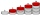What is the value of x2, x3, x4, x5…of the terms (of arithmetic progression) when x1 = 8 and x6 = 20?
• What areWhat are the four terms between 4 and 19?
• Two xeroxes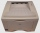The performances of the two copiers are in the ratio 3: 4. A machine with higher power will make 7,200 copies in one hour. How many copies will both machines make together in 5 hours?
• ComplaintsThe table is given: days complaints 0-4 2 5-9 4 10-14 8 15-19 6 20-24 4 25-29 3 30-34 3 1.1 What percentage of complaints were resolved within 2weeks? 1.2 calculate the mean number of days to resolve these complaints. 1.3 calculate the modal number of day
• Concrete columnThe concrete column of the highway bridge has the shape of a block with dimensions of 1m x 0.8m x 25m. It should be lifted by crane to a height of 20m. What is the power of his engine if the lifting takes 2 minutes?
• Divisible by nineHow many three-digit natural numbers in total are divisible without a remainder by the number 9?
• Railway embankmentThe railway embankment section is an isosceles trapezoid, the sizes of the bases of which are in the ratio 5: 3. The arms have a length of 5 m, and the height of the embankment is 4.8 m. Calculates the size of the embankment section area.
• Original planGardeners planted 16% more currant bushes than originally planned. In total, they planted x = 435 shrubs. What was the original plan?
• The pyramid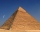The pyramid with a square base is 50 m high and the height of the sidewall is 80 m. Find the endge of the base of the pyramid.
• Tennis aces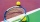The number of aces served by Novak Djokovic in the last 20 tournaments that he has participated in, is shown below. 12 17 13 7 8 14 11 14 10 12 15 9 11 13 6 15 18 5 19 24 1.1 using the raw, determine the range. 1.2 Group the data into a frequency distribu
• Pentagonal pyramidFind the volume and surface of a regular pentagonal pyramid with a base edge a = 12.8 cm and a height v = 32.1 cm.
• IQ Intelligence quotientIntelligence quotient (IQ) is a standardized score used as the output of standardized intelligence psychological tests to quantify a person's intelligence with the rest of the population (respectively to a given group). Intelligence has an approximately n
• Calculate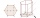Calculate the surface of a regular eleven-sided prism; if the area of its base is 58 cm2, the edge of the base is 6cm long, the height of the prism is 21 cm.
• Three colors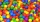Find the probability that 3 balls of the same color will be drawn from fate with 10 white, 10 red, and 10 blue balls.
• Coke and coal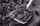20.1 tons of coke is produced from 30 tons of black coal. How much coke is made from 1 kilogram of coal?
• Assembly timeThe assembly time for the toy follows a normal distribution with a mean of 75 minutes and a standard deviation of 9 minutes. The company closes at 5 pm every day. If one starts assembling at 4 pm what is the probability that he will finish before the comp
• Alopecia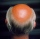Medical literature indicates that 45% of men suffer from alopecia. For random sample of 8 men, calculate the probability that: (a) exactly four men suffer from alopecia. (b) at most two men suffer from alopecia.
• Secondary school1/2 of the pupils want to study at the secondary school, 1/4 at the apprentice, 1/6 at the grammar school 3 pupils do not want to study. How many students are in the class?
• Convalescent homes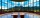In 270 convalescent homes, 94,270 vacationers spent part of the holiday a year. On average, how many vacationers per convalescent home?
• Isosceles trapezoidFind the area of an isosceles trapezoid with bases of 8cm and 72mm. The height of the trapezoid is equal to three-quarters of the longer base.

Do you have an interesting mathematical word problem that you can't solve it? Submit a math problem, and we can try to solve it.

We will send a solution to your e-mail address. Solved examples are also published here. Please enter the e-mail correctly and check whether you don't have a full mailbox.

Please do not submit problems from current active competitions such as Mathematical Olympiad, correspondence seminars etc...